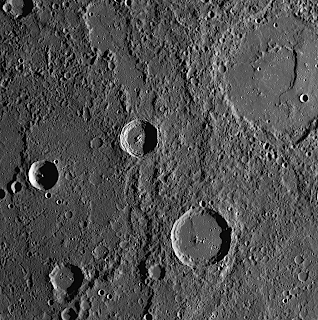## Monday, October 13, 2008

### CS: Fast and Efficient Dimensionality Reduction Using Structurally Random Matrices, SAMP code, Nuclear Norm Minimization using SRMsFollowing last week's entry that mentioned the connection between Random Projections used in CS and the ones used in Dimensionality Reduction based on the Johnson-Lindenstrauss Lemma, Thong Do pointed out to me his recently submitted paper entitled:

Fast and Efficient Dimensionality Reduction Using Structurally Random Matrices by Thong Do, Lu Gan , Yi Chen, Nam Nguyen and Trac Tran. The abstract reads:

Structurally Random Matrices (SRM) are first proposed in  as fast and highly efficient measurement operators for large scale compressed sensing applications. Motivated by the bridge between compressed sensing and the Johnson-Lindenstrauss lemma  , this paper introduces a related application of SRMs regarding to realizing a fast and highly efficient embedding. In particular, it shows that a SRM is also a promising dimensionality reduction transform that preserves all pairwise distances of high dimensional vectors within an arbitrarily small factor ², provided that the projection dimension is on the order of O(\epsilon^(-2) log^3 N), where N denotes the number of d-dimensional vectors. In other words, SRM can be viewed as the suboptimal Johnson-Lindenstrauss embedding that, however, owns very low computational complexity O(d log d) and highly efficient implementation that uses only O(d) random bits, making it a promising candidate for practical, large scale applications where efficiency and speed of computation are highly critical.

He is also hosting a webpage on the subject of Structurally Random Matrices (SRMs) I am now changing the attendant link of the Big Picture to that page.Sariel Har-Peled shows in ex 3.1 of this manuscript on the Johnson-Lindenstrauss lemma that one can also conserve angles as well as distance. Angle preservation was also the subject of interest of Jarvis Haupt and Robert Nowak in A generalized restricted isometry property. One wonders if the SRMs can do the same.

We mentioned SAMP before, Thong Do has released the attendant matlab code of the SAMP algorithm. It is listed in the reconstruction section of the Big Picture.

In a different area, Thong Do, Yi Chen, Nam Nguyen, Lu Gan and Trac Tran have also released A Fast and Efficient Heuristic Nuclear Norm Algorithm for Rank Minimization. The abstract reads:

The problem of affine rank minimization seeks to find the minimum rank matrix that satisfies a set of linear equality constraints. Generally, since affine rank minimization is NP-hard, a popular heuristic method is to minimize the nuclear norm that is a sum of singular values of the matrix variable . A recent intriguing paper  shows that if the linear transform that defines the set of equality constraints is nearly isometrically distributed and the number of constraints is at least O(r(m + n) logmn), where r and m £ n are the rank and size of the minimum rank matrix, minimizing the nuclear norm yields exactly the minimum rank matrix solution. Unfortunately, it takes a large amount of computational complexity and memory buffering to solve the nuclear norm minimization problem with known nearly isometric transforms. This paper presents a fast and efficient algorithm for nuclear norm minimization that employs structurally random matrices  for its linear transform and a projected subgradient method that exploits the unique features of structurally random matrices to substantially speed up the optimization process. Theoretically, we show that nuclear norm minimization using structurally random linear constraints guarantees the minimum rank matrix solution if the number of linear constraints is at least O(r(m+n) log3 mn). Extensive simulations verify that structurally random transforms still retain optimal performance while their implementation complexity is just a fraction of that of completely random transforms, making them promising candidates for large scale applications.

Credit: NASA/Johns Hopkins University Applied Physics Laboratory/Carnegie Institution of Washington, The First Image After Closest Approach of Fly-By 2 of Messenger at 200 kilometers above the surface, Release Date: October 8, 2008